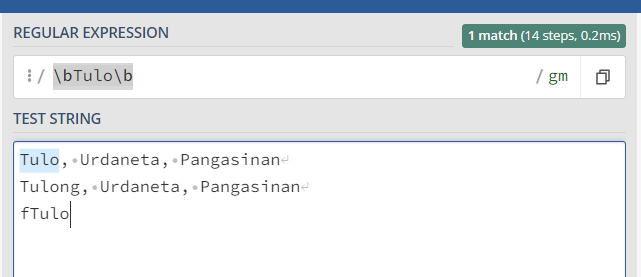# How to use contains to find the exact word?

How can I fix this?

The last cell value doesn’t match with the address data.

Is it possible to use contains and still get the output I’m expecting? If I use Equals, the output returns false since it doesn’t match.

I use this condition to look for the word I’m looking:

``````dtRef.AsEnumerable.Where(function(x) CurrentRowData("Address").ToString.Contains(x("Province").ToString)).Count > 0
``````

Then I write the data that matches using write cell

``````dtRef.AsEnumerable.Where(function(x) CurrentRowData("Address").ToString.Contains(x("Province").ToString)).Select(function(x) x("Province").ToString)(0)
``````

For reference:

AutoFilter.zip (3.6 KB)

Hi,

1 Like

@Shoji

You can try regex match

`dtRef.AsEnumerable.Where(function(x) System.Text.RegularExpressions.Regex.Match(CurrentRowData("Address").ToString,"([^a-zA-Z]|^)+" +x("Province").ToString + "([^a-zA-Z]|\$)+")).Select(function(x) x("Province").ToString).Count>0`

Hope this helps

cheers

1 Like

Hi @Shoji ,

Could you let us know for which data the word “Tulo” could be a match ?

In this way we could be more precise on what to suggest. A try could be done using the regex word boundary expression :

``````\bTulo\b
``````Modified Expression and a different appraoch :

``````dr_Array = dtRef.AsEnumerable.Where(Function(x) Regex.IsMatch(CurrentRowData("Address").ToString,"\b"+x("Province").ToString+"\b")).ToArray
``````

Here, `dr_Array` is DataRow array type variable.

Next, Using an `If` Condition Activity, we check if there is data in `dr_Array`, then we pick the First item matched.
Condition in `If` Activity :

``````dr_Array.Any
``````

In Then Block :

``````writeCellValueVar = dr_Array.First.Item("Province").ToString
``````

Else Block :

``````writeCellValueVar = ""      //Assign the value you would want to enter when there is no match
``````

The above expression reduces duplicacy of comparison code.

1 Like

Thanks for the comment, the word “tulo” belongs to a different address (Tulo, Taal, Batangas). I’ll try this one.

1 Like

Thanks I’ll try it

1 Like

Hello sorry for the late reply, I tried it but some doesn’t work well.

For example:

I guess, this is because in my reference sheet, there are “poblacion” but in different areas

I’m sorry if this is very confusing

hello sorry for late reply, I tried it but it’s not functioning well:

@Shoji

Can you share a input file and your reference file with some variations…that might help in suggesting a better one

Cheers

here’s the main file

AutoFilter - Copy.zip (159.2 KB)

here’s the excel file

ref.xlsx (858.9 KB)

Thank you

@Shoji

I did try with the file…and I fail to understand what is not proper…As the given regex identifies each string separately. Is that not the ask?

cheers

This topic was automatically closed 3 days after the last reply. New replies are no longer allowed.